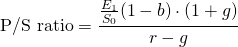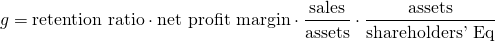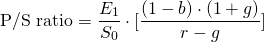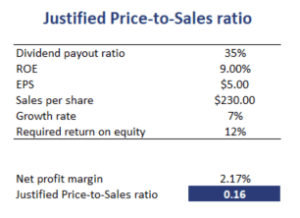# Justified Price-to-Sales ratio

The justified-price-to-sales ratio or justified P/S multiple is the price-to-sales ratio based on the Gordon Growth Model (GGM). Thus, it is the price-to-sales ratio based on the company’s fundamentals. This is different from the price-to-sales (P/S) ratio that is typically reported in financial media, which is based on historical sales and the observed stock price.

On this page, we discuss the justified price-to-sales ratio formula and illustrate how to calculate the ratio using a numerical example. The P/S ratio calculator can be downloaded at the bottom of the page

## Justified price-to-sales ratio formula

The formula for the justified P/S ratio is the followingwhere E0 are the earnings, S0 are the sales. The ratio of these two variables is the net profit margin. We see that the profit margin influences the justified P/S directly as well as indirectly. The indirect impact comes from the sustainable growth rate g, which depends on the retention rate bFinally, r is the required rate of return.

## Justified P/S interpretation

The formula indicates that the P/S ratio will go up, all else equal, if the profit margin increases and when the earnings growth rate increases. Interestingly, we can rewrite the formula such that the P/S ratio can be calculated using the trailing justified P/E.The second term is the justified trailing P/E ratio.

## Justified price-to-sales multiple example

If we have all the relevant data on a company, we can calculate the justified ratio and compare it to the observed P/S ratio. If the observed price-to-sales ratio is higher than the justified P/S ratio, than the stock is overvalued. If the justified price-to-sales is higher than the actual P/S, then the stock is undervalued and an analyst should consider issuing a buy recommendation.

The following table uses the above formula to calculate the justified P/S ratio. The spreadsheet used to create this example can be downloaded below the summary.## Summary

We discussed the justified P/S ratio. This measure can easily be calculated using data on the net profit margin and the retention ratio, growth rate and required rate of return.

### Justified P/S

Want to have an implementation in Excel? Download the Excel file: Justified Price-to-sales